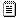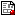[ Data Screening || Matrix Algebra with SAS/IML || Regression analysis || General Linear Models || Canonical correlation, Discriminant analysis || Logistic Regression || Factor Analysis || Clustering and scaling || SAS macro programs || Data sets || Programs]

## Regression examples

These tutorial examples use a few different data sets to illustrate a variety of methods involved in regression analysis.
autocox.sasBox-Cox transformation for the Auto data. Uses the BOXCOX macro to find a power transformation of MPG.
basecox.sasBox-Cox transformation for the Baseball data. Uses the BOXCOX macro to find a power transformation of Salary.
bisqdunc.sasRobust Regression - Duncan data. Uses the BISQUARE macro.
bisqfuel.sasRobust Regression - Fuel data. Uses the BISQUARE macro.
bloodreg.sasTesting homogeneity of regression. Compares models with different slopes and intercepts in the BLOOD data.
bootdunc.sasBootstrap Regression - Duncan data. Uses the BOOT macro.
crossval.sasPlot of predictive R-square, showing effects of shrinkage.
detroit1.sasPredicting Detroit homicide rates. See the description of DETROIT.SAS under Data sets.
detroit2.sasStepwise selection methods on Detroit Homicide data.
evappca.sasIncomplete principal components regression.
fitbipl.sasFitness data: Biplot.
fitcp.sasFitness Data: CP Plot.
fitcolin.sasFitness Data: Collinearity diagnostics.
fitinfl.sasFitness Data: Influence plot, bubble proportional to Cook's Distance
fitness2.sasInfluence diagnostics and partial residual plots
fitness3.sasVarious selection methods for Fitness data
fitness4.sasCross validation of a regression model. We hold back a portion of the data from the fit, and evaluate how well the model predicts in the hold-back sample.
fitpart.sasFitness data: Partial residual plots.
fitstep.sasIllustrate stepwise selection using the Fitness Data.
fuelcox.sasFuel Consumption: Box Cox Transformation Plots.
fuelcp.sasC(p) and related plots for fuel data.
fuelerr.sasFuel Consumption: Demonstrates effects of errors-in-predictors.
fuelinfl.sasFuel Consumption: Influence Plot. Uses the INFLPLOT macro to show the influence of a few observations on prediction of fuel consumption.
fuelpart.sasFuel data: Partial residual plots
fuelr1.sasFuel data: Building a regression model
health.sasSuppression effects: Health, Height and Weight
mregppvt.sasMultivariate multiple regression
regdemo.sasRegression example, using SAS/IML. A set of SAS/IML modules to carry out regression computations and hypothesis tests. Duplicates PROC REG facilities, while the IML program shows how it's done.
reginfl.sasInfluential Observations in Multiple Regression. Fictitious data to illustrate various combinations of residual and leverage to produce influential observations.
robdunc.sasRobust Regression - Duncan data, using the ROBUST macro.
robfuel.sasBisquare Robust Regression - Fuel data, using the ROBUST macro.
robreg.sasRobust Regression using IRLS(via PROC NLIN; superceded by ROBUST macro).
scatfuel.sasFuel data: scatterplot matrix
stepsim.sasStepwise regression simulation example NO real predictors. The maxim if you torture the data long enough, you can make it confess to anything is illustrated by constructing 100 random predictors, all statistically independent of the response. How many do you suppose will be significant at the .05 level?
stepsim2.sasStepwise simulation experiment: add random predictors to Fitness Data. What do you think happens if you throw a whole bunch of random predictors into stepwise regression with a real data set?
suppress.sasDemonstrate suppression effect. Fictitious data on three variables are used to illustrate a situation in which an additional variable can make a greater extra contribution to regression, SSR(X2|X1), than it does by itself, SSR(X2).
suppres2.sasPartial residual plots for supression example
therboot.sasSimple Regression: Bootstrap samples and estimates
turnip.sasLurking variables: Turnip Green Data. Plots of results from a model to predict the quantity of Vitamin B2 in turnip greens reveal a surprising and unsuspected lurking variable.